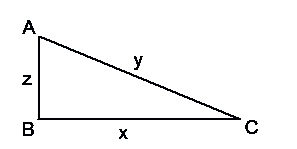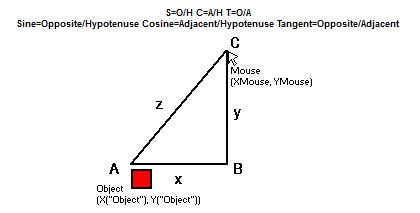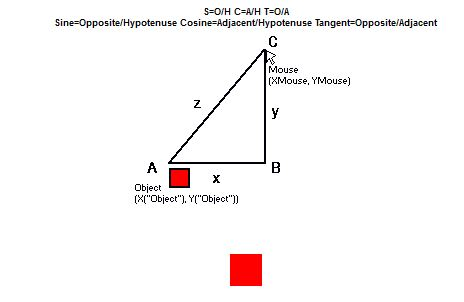# Trigonometry In Gaming

So you want to do some cool effects in your game; some full 360 degree shooting, maybe some sine waves, and maybe spinning objects.  With normal 32-directional MMF2 movements, this is not possible.  You can get accurate, but you're not going to get anywhere near the nice, professional movements that you can easily learn.

### Introduction

I'm not asking you for much time, this is a very simple tutorial, and I'll explain it all.  Please, if you're planning on learning 360 shooting or trigonometry, continue reading.  You will not find a shorter method, so here, just waste your 15 minutes and be done.  To learn, you must have some time to spare.

We will cover, in this tutorial, Sine, Cosine, Tangent, and Arc Tangent.  We will not need to use any of the other trigonometric functions.

Basic understanding of MMF2 is needed for this tutorial.  If you find it hard to understand the actions and how we control our objects, perhaps this tutorial is not right for you.  This is a somewhat advanced tutorial, for those who want to have more power and control in their games.
Now if you have problems understanding the mathematics and expressions, then don't just quit, try to understand.  Find me, Eliyahu, and I'll help you out.

Now that the intro is done....TO THE LEARNING!

### Triangle

Consider the right triangle below, ABC.  It's angles are A, B, and C, and its sides are x, y, and z. Normally sides would be labelled the lowercase letter of their opposing angle, but for ease of understanding, we will use x, y, and z:Now, on a slightly different note, consider the text, SOHCAHTOA.  This little "word" will always be important, as it describes the relationships between angles and 2 sides.

• S=O/H C=A/H T=O/A

You should already know that hypotenuse is the longest side of a right triangle, the side opposite the right angle.  a^2+b^2=c^2, the Pythagorean Theorem, should be review to you, so we will skip over that. Opposite, is as stated, the side opposite an angle.  The opposite of C is z.  Adjacent is the side that is next to, or touching an angle, but NOT the hypotenuse.  The adjacent side to C is x.

Keeping in mind the above, we can find a lot out about an angle. For example: (if you didn't realize it, Cos=cosine, Sin=sine, Tan=tangent)

• Cos(A) = z/y
• Cos(C) = x/y
• Sin(A) = x/y
• Sin(C) = z/y
• Tan(A) = x/z
• Tan(C) = z/x

And don't even bother trying to use trigonometric functions on the right angle, B, it doesn't work. This may be a lot to take all at once, but it is in fact, very simple.  You have your set of rules, SOHCAHTOA, so if you want to find the sine, cosine, or tangent of an angle, you can do so!

NOTE: You don't need to memorize those functions pertaining to our triangle, because with SOHCAHTOA, you can find them at any time.  The key is SOHCAHTOA, and you can find it for ANY right triangle.

### Atan2

Now that we have a basic understand of the 3 main trigonometric functions, lets take a look at Arc Sine, Arc Cosine, and Arc Tangent. Let's say we have 2 side of a triangle, now we want the angle.  Before, we would take the angle, perform the function on it, and get a number out of it.

• Consider: Cos(A)=z/y

Given z and y, we can say that the angle, A, can be found when the cosine equals z/y.  We do this with Arc Cosine.  It is noted as ACos (ASin, and ATan, as well).  For example, you would solve it as:

• A = ACos(z/y)

This says, let A equal the value when the cosine of that value is equal to z/y.For gaming, we only need to worry about ATan right now, but we will get to that.  To keep your mind in line with this, remember the following, pertaining to the triangle above:

• C = ATan(z/x)

This will come into play soon...  But now let's focus on implementing this into a game.

### Mouse Triangle360 degree shooting is the most common occurence of trig in gaming.  So imagine the X and Y positions of an Object, and of your mouse.  We want to shoot an object in that direction. It is understood that x = Xmouse-X("Object") and y = Ymouse-Y("Object") Together they create a triangle, with z being the hypotenuse. If this is moving too fast and you are confused, don't go farther, it will only get more confusing.  I apologize, I'm doing the best I can.

So we want to shoot a projectile from point A in the direction of C.  It is evident that we need the angle A to go any farther.  It could be done with C, but A is a smarter, clearer choice, so let's get angle A. But how?  All we have are x and y, which are the differences in the x and y position of our mouse in comparison to the object. BUT we can use ATan to obtain angle A.

Think about it, the tangent of angle A is y/x.  Therefore, A = ATan(y/x).  This seems fine and dandy, and it should be, but MMF2 and other languages will let us use ATan2, which is the only option for 360 shooting, so lets look at ATan2.

In MMF2 we have access to all of the normal functions, ACos, ASin, ATan, and ATan2. Eliyahu, what is this ATan2?!?! Well, ATan only returns the angle in a range of -90 to 90.  This is 180 degree movement.  That won't suffice for a full rotational shooter.  So we use ATan2, which has a range of -180 to 180, the full 360 degrees of a circle!

Forget ATan, you won't need it, really.  If you ever can't decide which, use ATan2. So we determined that the angle, A is actually equal to ATan2(y/x).  The problem is that MMF2 takes the parameter as a y and an x parameter.  This means that you have 2 separate values to enter, the numerator of the fraction, and the denominator.  This is so it can determine the angle, as tangent cannot possibly specify the 360 degrees, so MMF2 uses logic of positives and negatives in direction to determine what angle it will be.  If you study the unit circle, you will understand, but you do not need to at this point, and it is not included in this tutorial. To sum it all up, the angle A = ATan2(YMouse-Y("Object"), XMouse-X("Object")) It would normally be y/x, but as we said, we need the numerator and denominator as 2 separate parameters.

We know angle A.  But what good does that do us if we can't move in that angle?  So let's cover up movement in that direction. What we are really looking for are x and y, one at a time, to figure out how much they will move in that and x and y axis.  Consider z to be the length of the movement.  It is the hypotenuse and therefore overall amount of pixels travelled.  If we forget to put that in our expression, it will be assumed 1, and then the x and y will be less than or equal to 1 and our object will go nowhere.

Given A and z, let's find x.  We know that Cos(A) = x/z Multiply both sides by z, and we find x = z*Cos(A) It is that simple.  We can do the same for y.  Sin(A) = y/z, therefore y = z*Sin(A) Let's be easy and let z=10.  For this tutorial, on creation of the bullet or projectile, we store the angle in an alterable value, 'rot'. (rotation) We will add to the X position 10*Cos(rot("Bullet")), and to the Y Position, 10*Sin(rot("Bullet")) Let's see what this looks like!  In other words, we finally get to see some action!

### 360 Degree shootingThe red object, Base, is somewhat centered.  It creates objects, Bullets.  Look at the code, to see how it works.  (SEE DOWNLOAD BELOW) Click to shoot. Next, we will make it a little more dynamic. We will also clean it up a little, because you'll notice that things aren't centered, and the bullet ends up being slightly off where you want it to go.

This time, the Bullet has an alterable value, 'vel' (velocity) that it will travel.  We can change this, because it is a variable.  We are not longer limited to 10!
If you hold down the left mouse button, all the existing bullets will slow down.  We could have them initialize at this speed from a global value that they all look at upon creation, but let's keep it simple. Holding down the right button will add to the rotation, so they will curve.

DEMO FRAME AVAILABLE IN MFA

### Orbit like a satellite with Trigonometry

Let's go over what we can do with trigonometry so far: We can determine an angle given an x and y difference. We can determine x and y movement when given an angle and speed.

Let's look at that second one.  So far we've used angle A for the angle, but what if we used an unrelated angle?  An angle that always increases.  We can create a satellite, an object that circles around another.

The center of our satellite is (x,y) which can also be (X("Object"), Y("Object")) or (XMouse, YMouse). If our satellite has an alterable value rot that we continually add onto, we can set its X position to be X+75*Cos(rot("satellite")) and Y to Y+75*Sin(rot("satellite")) then we will have an orbitting object 75 pixels in radius from the center, (X,Y).

Let's take a look at how that turns out, below.  Comments explain what's going on. The next frame will be slight changes to this example.

DEMO FRAME AVAILABLE IN MFA

### Sine & Cosine

Let's step back a bit, and look at Sin(x). You don't need to understand the unit circle quite yet, unless you want very specific waves.  For now, you can understand the following....you don't even need to know this.  Just know how a sine wave reacts:

• Sin(0)=0
• Sin(45)=sqrt(2)/2
• Sin(90)=1
• Sin(135)=sqrt(2)/2
• Sin(180)=0,
• Sin(225)=-sqrt(2)/2
• Sin(270)=-1
• Sin(315)=-sqrt(2)/2
• Sin(360)=0

This will repeat even after you go above 360.  360==0 in angles, so 405==45.  Adding onto the angle will just work in a loop.  Below is a series of dots, where they arranged in a sine wave.  Refer to the comments for more detail on how they are set up. Also, keep in mind that Sin(x) will always be between -1 and 1, so you need to mutliply by another number so you see the result, not a range of 2 pixels.

Now that we saw a sine wave, we can look at cosine!  It is very similar:

• Cos(0)=1
• Cos(45)=sqrt(2)/2
• Cos(90)=0
• Cos(135)=-sqrt(2)/2
• Cos(180)=-1,
• Cos(225)=-sqrt(2)/2
• Cos(270)=0
• Cos(315)=sqrt(2)/2
• Cos(360)=1

This as well, repeats, like sine.  The blue dots shown below are cosine, in comparison to the red sine dots. Next we will make the wave a lot more dynamic, with direct input for all the controls.

DEMO FRAME AVAILABLE IN MFA

Original Author: Eliyahu